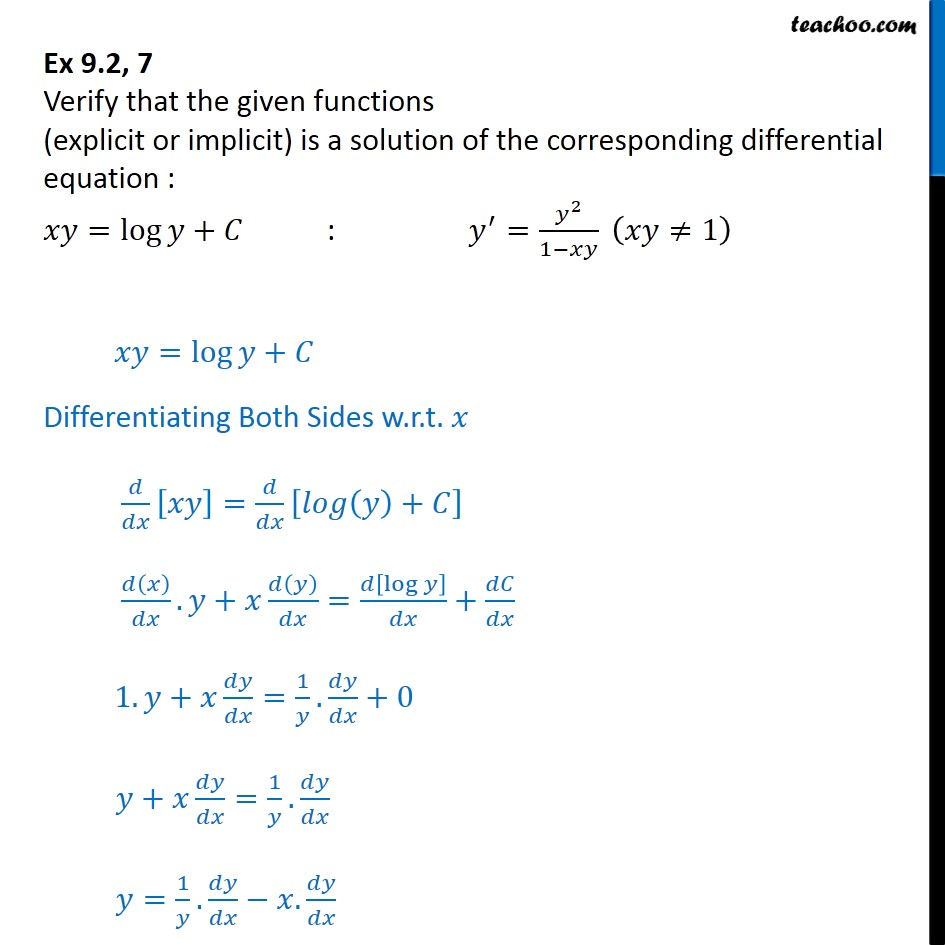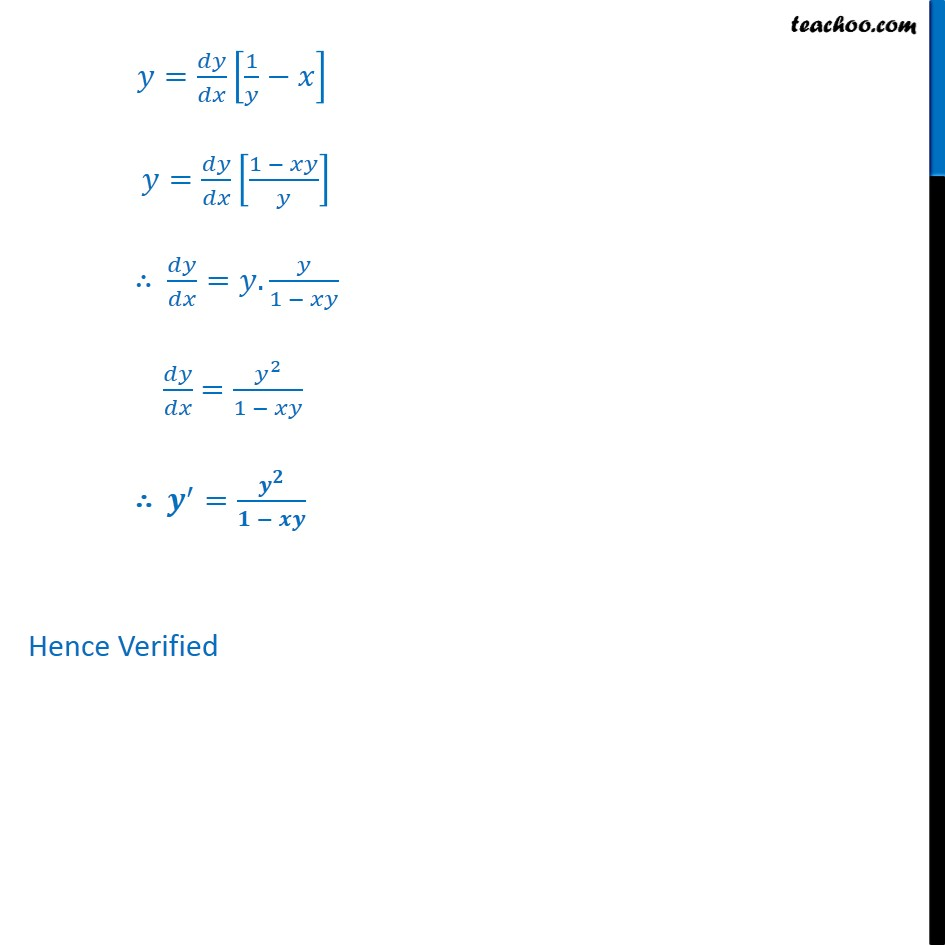Ex 9.2

Chapter 9 Class 12 Differential Equations
Serial order wiseLearn in your speed, with individual attention - Teachoo Maths 1-on-1 Class

### Transcript

Ex 9.2, 7 Verify that the given functions (explicit or implicit) is a solution of the corresponding differential equation : = log + : = 2 1 1 = log + Differentiating Both Sides w.r.t. = + . + = log + 1. + = 1 . +0 + = 1 . = 1 . . = 1 = 1 = . 1 = 2 1 = Hence Verified